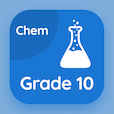Engineering Online Courses

Engineering Physics Quizzes

Engineering Physics Quiz PDF - Complete

# Melting Points Quiz MCQ Online p. 114

Practice Melting Points quiz questions and answers PDF, melting points trivia questions 114 to learn online Engineering Physics course for online classes. Properties of Common Elements MCQ questions, Melting Points Multiple Choice Questions (MCQ) for online college degrees. "Melting Points Quiz" PDF eBook: ohm law, celsius and fahrenheit scales, collision and impulse, spherical mirror, melting points test prep for associate degrees in engineering.

"637°C is melting point of" MCQ PDF: nitrogen, neptunium, terbium, and selenium for online engineering graduate colleges. Solve properties of common elements questions and answers to improve problem solving skills for free career quiz.

## Trivia Quiz on Melting Points MCQs

MCQ: 637°C is melting point of

Neptunium
Nitrogen
Terbium
Selenium

MCQ: Mirrors used in reflecting telescopes, catadioptric lens systems and headlamps are

plane mirrors
concave mirrors
convex mirrors
virtual mirrors

MCQ: Measure of both magnitude and duration of collision force is termed as

impulse
momentum
work done
kinetic energy

MCQ: Freezing point of water in Fahrenheit is

98.6°F
212°F
32°F
68°F

MCQ: Law which states that current through the device is always directly proportional to the potential difference applied to the device is called

Ohm's Law
Newton's Law
Leibniz's law

### More Quizzes from Engineering Physics Course